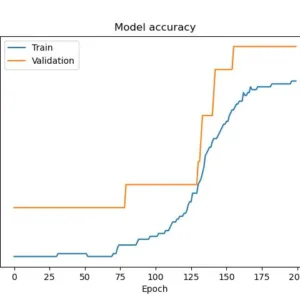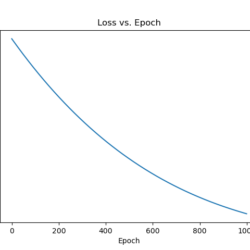## Logistic Regression in TensorFlow

Logistic Regression is a popular machine-learning algorithm that is used for classification tasks. In this tutorial, we will learn how to implement Logistic Regression in TensorFlow 2.0…